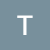# Multi-class Classification — One-vs-All & One-vs-One

Statistical models such as Perceptron, Logistic Regression and Support Vector Machine are designed to classify two classes at a time and do not natively support classification tasks with more than two classes.

So, to Implement multiclass classification in the above models, We usually split the multi-class classification dataset into multiple binary classification datasets and fit a binary classification model on each. This leads to two different meta strategies — OVR and OVO

One vs Rest (OVR)

OVR strategy splits a multi-class classification into one binary classification problem per class.

For eg- If we want to classify Red, Blue and Green

# Splitting Criteria and Algorithms in Decision Tree

Decision Tree is a type of supervised learning algorithm that can be used in both regression and classification problems.

Goal in Decision Tree

Goal in Decision tree is to create a training model which can be used to predict the value or the class of the target variable by learning simple decision rules and terminologies inferred from a training data.

Idea behind Decision Tree

At each point or a training sample, We consider a set of questions that can partition our dataset.

We choose the question that provides the best split and again find the best question for the partition.

# Support Vector Machine

SVM ( Support Vector Machines ) is a supervised machine learning algorithm which can be used for both classification and regression challenges. But, It is widely used in classification problems.

In SVM, we plot each data item as a point in n-dimensional space (where n = no of features in a dataset) with the value of each feature being the value of a particular coordinates. Such that, value of feature is equal to the value of coordinate then we perform classification by finding the most appropriate hyperplane that differentiates two classes very well.

# Goal in SVM

Our goal is to determine some establishing…